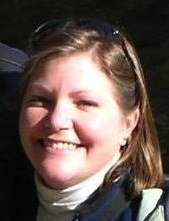Home

Teaching

Research

Matlab

Links

Below is a list of the courses I've taught at Loyola University Maryland. Following is a history of the courses organized by semester and by course.

## List of Courses

• FE100/LOY101: First-year Experience
• GB500: Math Fundamentals for Business
• MA109: Precalculus
• MA151: Applied Calculus
• MA151: Applied Calculus online course
• MA251: Calculus I
• MA252: Calculus II
• MA/CS295: Discrete Structures
• MA301: Intro. to Linear Algebra
• MA302: MATLAB Laboratory (1-credit)
• MA302: Programming in Mathematics (3-credit)
• MA351: Calculus III
• MA395: Discrete Methods
• MA402: MATLAB Programming in Mathematics
• MA421: Analysis I
• MA422: Analysis II
• MA424: Complex Analysis

## By Semester (most recent first)

NOTE: some course information may no longer be available, and additional information may be on Moodle.

Fall 2018
MA421: Analysis I
Summer 2018
MA151: Applied Calculus (online)
Spring 2018
MA151: Applied Calculus - Messina program
MA302: Programming in Mathematics
Fall 2017
MA251: Calculus I
Summer 2017
MA151: Applied Calculus (online)
Spring 2017
MA151: Applied Calculus - Messina program
Fall 2016
MA251: Calculus I
MA421: Analysis I
MA424: Complex Analysis (private study)
Spring 2016
MA302: Programming in Mathematics
MA395: Discrete Methods
MA421: Analysis I (private study)
Fall 2015
MA351: Calculus III
MA421: Analysis I
Spring 2015
MA301: Intro. to Linear Algebra
MA395: Discrete Methods
Fall 2014
MA351: Calculus III
Loyola 101
Spring 2014
None: on sabbatical.
Fall 2013
MA251: Calculus I
MA402: MATLAB Programming in Mathematics
LOY101 (formerly FE100: First-year Experience)
Spring 2013
MA252: Calculus II
MA424: Complex Analysis
Fall 2012
GB500: Fundamentals of Math for Business
MA151: Applied Calculus
MA402: MATLAB Programming in Mathematics
FE100: First-year Experience
Summer 2012
GB500: Fundamentals of Math for Business
Spring 2012
MA301: Intro. to Linear Algebra
MA402: MATLAB Programming in Mathematics
Fall 2011
MA351: Calculus III
MA402: MATLAB Programming in Mathematics
MA424: Complex Analysis (private study)
FE100: First-year Experience
Spring 2011
MA301: Intro. to Linear Algebra
MA424: Complex Analysis
Fall 2010
MA252: Calculus II
MA302: MATLAB Laboratory
FE100: First-year Experience
Spring 2010
MA301: Intro. to Linear Algebra
Fall 2009
None: on family leave
Spring 2009
MA251: Calculus I
MA424: Complex Analysis
Fall 2008
MA302: MATLAB Laboratory
MA351: Calculus III
FE100: First-year Experience
Spring 2008
MA252: Calculus II
MA422: Analysis II
MA422 (private study)
Fall 2007
MA302: MATLAB Laboratory
MA351: Calculus III
MA421: Analysis I
Spring 2007
MA252: Calculus II
MA424: Complex Analysis
Fall 2006
MA302: MATLAB Laboratory
MA351: Calculus III
Spring 2006
None: on sabbatical
Fall 2005
MA251: Calculus I
FE100: First-year Experience
Spring 2005
MA252: Calculus II
MA424: Complex Analysis
Fall 2004
MA302: MATLAB Laboratory
MA351: Calculus III
MA421: Analysis I
Spring 2004
MA301: Intro. to Linear Algebra
MA422: Analysis II
MA424: Complex Analysis (private study)
Fall 2003
MA251: Calculus I
MA421: Analysis I
MA424: Complex Analysis (private study)
FE100: First-year Experience
Spring 2003
MA251: Calculus I
MA424: Complex Analysis
Fall 2002
MA 109: Precalculus
MA/CS 295: Discrete Structures

## By Course

ClassSemester
FE100/LOY101F03, F05, F08, F10, F11, F12, F13, F14
GB500Su12, F12
MA109F02
MA151F12, S17m, S18m
MA151 onlineSu17, Su18
MA251S03, F03, F05, S09, F13, F16, F17
MA252S05, S07, S08, F10, S13
MA/CS295F02
MA301S04, S10, S11, S12, S15
MA302 (1-credit)F04, F06, F07, F08, F10
MA302 (3-credit)S16, S18
MA351F04, F06, F07, F08, F11, F14, F15
MA395S15, S16
MA402F11, S12, F12, F13
MA421F03, F04, F07, F15, S16p, F16, F18
MA422S04, S08, S08p
MA424S03, S05, S07, S09, S11, S13
F03p, S04p, F11p, F16p

m: taught within Messina program
p: taught course as private study